• Call Now

1800-102-2727•

# Resonance - Free, Damped and forced oscillation, practice problems, FAQs

Have you ever swung on a playground? The swing begins to go forward and backward when we push it. The motion of the swing can be developed by giving it a series of pushes. If the person pushing the seat matches the timing of the swing as he applies the force, the swing's action has a greater amplitude and can therefore swing higher. This is the condition of resonance. Even a light push on the swing helps to sustain its amplitude due to the resonance. This happens as it has reached its natural frequency of oscillation. This coordinated motion is known as resonance. Let's understand the resonance in detail.

Table of content

## Free, Damped and forced oscillation

Free oscillation occurs when a system oscillates at a frequency that is unique to its geometry and inertia, also referred to as its natural frequency. It is an ideal situation. In free oscillation, frequency, amplitude and the energy of oscillation remain constant. Motion of the spring block system in a non resisting medium is an example of free oscillation.When oscillations occur in such a way so that their energy is faded out due to some external force acting on it, it is called damped oscillation. In this oscillation the amplitude of the oscillation decreases with time and after some time it reduces to zero. Damped oscillations make up every practical oscillation.

The forced oscillation is the oscillation that occurs when a body oscillates under the influence of an external periodic force. This external periodic force makes up for the energy which is lost because of the damping force. So the oscillation's amplitude remains constant. Oscillation of the swing when someone is pushing periodically is an example of forced oscillation.

## Resonance

In forced oscillation the external force is periodic in nature and has some periodic frequency. When the frequency of the external force is changed the amplitude of the oscillation also changes. When the frequency of the external force is equal to the natural frequency of the oscillator or the system, the amplitude of the oscillation increases. This condition is referred to as the resonance state and this frequency is referred to as the resonant frequency.

Soldiers are instructed not to cross a bridge by marching, a singer is breaking a wine glass, radio, cooking food in a microwave oven etc. are some examples of resonance.

Now, let's see the resonance in a mathematical way.

## Amplitude of forced oscillation

Consider a spring-block system that performs free oscillation with a natural frequency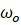and has a block of mass m and a spring of force constant k.

The block is moved away from its mean position. If the block's displacement at time t is x(t), then the spring's restoring force is,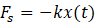A negative sign indicates that the force opposes displacement in the situation.

Now the system is placed in a damping medium of damping constant b as shown in figure.If the velocity of the block at time t is v(t), then the damping force acting on the system is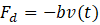An external periodic force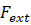is applied to a system whose frequency is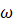and amplitude is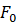which is given as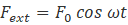The net force on the block is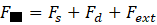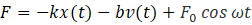If the acceleration of the block at any time t is equal to a(t), then we can write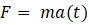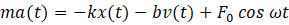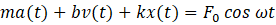On Substitute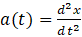and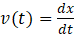, we have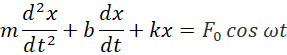The above differential equation represents the motion of a block under the combined action of restoring force, damping force, and driving force. The motion of forced oscillation will be given by solving this differential equation.

Displacement of the particle of the forced oscillation motion is given by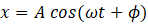Where,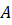is the amplitude of motion and given as,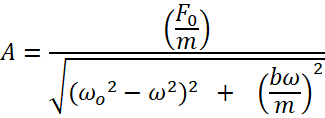is the frequency of force,is the natural frequency of oscillation and t is time measured from the instant of force application and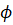is the initial phase.

Now consider the following cases -

1. When driving frequencyis much less than the natural frequencyof the system

(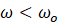), then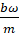can be neglected. Hence the amplitude is given as ,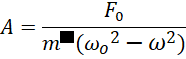The amplitude will depend on the difference in square of frequencies.

1. When frequency of force is close to natural frequency (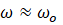) then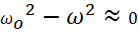Then amplitude is given as,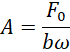Amplitude depends on the frequency of force.

1. When b=0 and the frequency of the external force () is equal to the natural frequency (),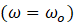then the amplitude of the oscillation is infinite. This state of oscillation is known as the state of resonance and this frequency is called the resonant frequency. Ideally the amplitude of oscillation at the time of resonance is infinite but for calculation purposes we can take the amplitude as,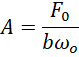The figure below shows the amplitude of oscillation with respect to the frequency for different values of damping.## Practice problems

Q. A block with a mass of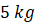is oscillating at a natural frequency of 20 rad/s. Driving force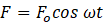, is applied to it, where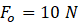and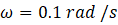. Find the amplitude of the oscillation.

A.Given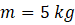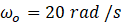As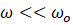, so the amplitude of oscillation is given as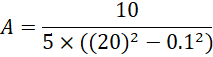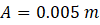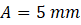Q. A periodic force of maximum value 50 N is applied on a spring block system of mass 30 kg and spring constant 100 N/m. If the damping constant of the motion is 40 kg/s, find the amplitude of resonating oscillation.

A. Mass, m = 30 kg

Damping constant, b = 40 kg s−1

Maximum force,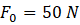Spring constant, k = 100 N m−1

The natural frequency of the system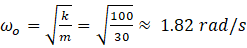At resonance the frequency of external force will be equal to natural frequency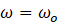The amplitude of oscillation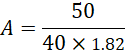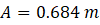Q. The amplitude of a forced oscillatory motion at resonance is 10 mm when it is placed in the medium of damping constant 0.5 kg/s. Find the amplitude of oscillation when it is placed in the medium of damping, constant 0.3 kg/s.

A.Given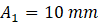and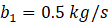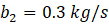The amplitude of oscillation at resonance condition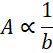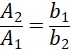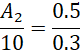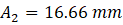Q.A spring of force constant 50 N m−1 is attached to the block of mass 500 gm. At what angular frequency of external force the system will start resonating?

A.GivenMass, m = 500 gm

Spring constant, k = 50 N m−1

At resonance the frequency of external force will be equal to the natural frequency of oscillation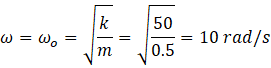## FAQs

Q1.How many types of resonance are there?
There are five types of resonance -they are Acoustic, Mechanical, Electrical, Optical, Orbital resonance.

Q2. What is a system's natural frequency?
Answer: The oscillation frequency of a system in the absence of any driving force or dampening is known as its natural frequency.

Q3.When the driving frequency is far from the natural frequency, what is the expression for the forced oscillator's amplitude?
Answer: When the driving frequency is far from the natural frequency the amplitude of the oscillation is given byWhere,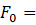force amplitude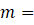mass of oscillator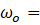Natural frequency of oscillator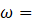frequency of external force

Q4.What happens to the oscillations' amplitude when the driving frequency approaches the oscillator's native frequency?
Answer:  The oscillation's amplitude increases and the system is considered to be in resonance when the driving frequency approaches the oscillator's native frequency.

Q5.What is the effect of damping on amplitude of oscillation at resonance?
Answer: Amplitude is inversely proportional to the damping constant. So if damping increases, the amplitude of oscillation decreases, and if damping decreases, the amplitude of oscillation increases.Talk to our expert
Resend OTP Timer =
By submitting up, I agree to receive all the Whatsapp communication on my registered number and Aakash terms and conditions and privacy policy